# Kurt GÃ¶del. Rotating Universes In General Relativity Theory .pdf

### File information

This PDF 1.5 document has been generated by TeX / MiKTeX pdfTeX-1.40.20, and has been sent on pdf-archive.com on 09/11/2019 at 16:05, from IP address 92.75.x.x. The current document download page has been viewed 510 times.
File size: 283.92 KB (11 pages).
Privacy: public file

### Document preview

ROTATING UNIVERSES IN
GENERAL RELATIVITY THEORY
KURT GÖDEL
(April 28, 1906 – January 14, 1978)

In this lecture I am setting forth the main results (for the most part without proofs)
to which my investigations on rotating universes have led me so far.

1

1 Definition of the type of rotatory
solutions to be considered
I am starting from the relativistic field equations: 1
Rik −

1
gik R = Tik − λ gik
2

(1.1)

and I am assuming that:
1. the relative velocity of masses (i.e. galactic systems) close to each other is small
compared with c.
2. no other forces except gravitation come into play.
Under these assumptions Tik takes on the form:
Tik = ρ vi vk

(1.2)

ρ &gt; 0,

(1.3)

where

ik

g vi vk = −1

(1.4)

The signature of gik is +2.

(1.5)

and, of course:

The local angular velocity of matter relative to the compass of inertia can be represented
by the following vector ω (which is always orthogonal on v):
ωi =

 iklm
aklm
12 (−g)1/2

where the skew-symmetric tensor aklm is defined by:






∂ vm
∂ vm
∂ vk
∂ vk
∂ vl
∂ vl

+ vl ·

+ vm ·

.
aklm = vk ·
∂ xm
∂ xl
∂ xk
∂ xm
∂ xl
∂ xk

(1.6)

(1.7)

That ω represents the angular velocity relative to the compass of inertia is seen as follows:
In a coordinate system which, in its origin, is geodesic and normal, and in whose origin
1

I am supposing that such measuring units are introduced as make c = 1, 8 π κ/c 2 = 1.

2

1 Definition of the type of rotatory solutions to be considered
matter is at rest (i.e. for which in O : ∂ gik /∂ xl , gik = ηik , v 4 = 1, v i = 0 for i 6= 4) 2 ,
one obtains for ω i in O:

 3
 2 
 3

1
∂ v2
1
v

v
∂v

ω1 = ·

= ·

, etc.
(1.8)
2
∂ x2
∂ x3
2
∂ x2 v 4
∂ x3 v 4
ω4 = 0 .

(1.9)

In such a coordinate system, however, since parallel-displacement (in its origin) means
constancy of the components, the angular velocity relative to the compass of inertia, in
O, is given by the same expressions as in Newtonian physics, i.e. the right-hand sides of
equation (1.8) are its components. Evidently ω is the only vector the first 3 components
of which, in the particular coordinate systems defined, coincide with the angular velocity
computed as in Newtonian physics and the 4th component is 0.
Any Riemann 4-space with some ρ, vi defined in it, which everywhere satisfies the conditions (1.1)-(1.5) and permits of no extension free from singularities, and for which,
moreover, ω is continuous and 6= 0 in every point, represents a rotating universe. However, in the sequel I am chiefly concerned with solutions satisfying the following three
further postulates (suggested both by observation and theory):
I. The solution is to be homogeneous in space (i.e. for any two world lines of matter
l, m there is to exist a transformation of the solution into itself which carries l into
m).
II. Space is to be finite (i.e. the topological space whose points are the world lines of
matter is to be closed, i.e. compact).
III. ρ is not to be a constant.
Postulate III is indispensable also for rotating universes, since it can be proved that a redshift which, for small distances, increases linearly with the distance implies an expansion,
no matter whether the universe rotates or not. 3
As to the question of the existence of rotating solutions satisfying the postulates I, II,
III, cf. §5.

2

3

A coordinate system satisfying the first two conditions may fittingly be called a ”local inertial
system”.
Provided, of course, that the atomic constants do not vary in time and space, or, to be more exact,
provided that the dimensionless numbers definable in terms of the constants of nature (such as
e 2 /h c) are the same everywhere.

3

2 Some general properties of these
solutions
In view of III the equation ρ = const. defines a one-parameter system of 3-spaces. In
rotating universes these 3-spaces of constant density cannot be orthogonal on the world
lines of matter. This follows immediately from the fact that aklm = 0 is the necessary
and sufficient condition for the existence of any system of 3-spaces orthogonal on a vector
field v.
The inclination of the world lines of matter toward the spaces of constant density yields
a directly observable necessary and sufficient criterion for the rotation of an expanding
spatially homogeneous and finite universe: namely, for sufficiently great distances, there
must be more galaxies in one half of the sky than in the other half.
In the first approximation, i.e., for solutions differing little from one spatially isotropic,
the magnitude of this effect is given by the following theorem: If N1 , N2 are the numbers of galaxies in the two hemispheres into which a spatial sphere 1 of radius r (small
compared with the world radius R) is decomposed by a plane orthogonal on ω, then:
|N1 − N2 |
9 |ω| r R h
= ·
N1 + N2
8
c2

(2.1)

where h is Hubble’s constant (= Ṙ/R).
For plausible values of the constants (where ω is estimated from the velocity of rotation
of the galaxies 2 ) this effect is extremely small. But the uncertainty in the knowledge of
the constants is too great for drawing any definitive conclusions.
The group of transformations existing owing to I evidently carries each of the spaces
ρ = const. into itself, and therefore (the case of isotropy being excluded) can only have
3 or 4 parameters 3 . The number 4 (i.e., the case of rotational symmetry) cannot occur
either. There exist no rotationally symmetric rotating universes satisfying the conditions
stated in §1 4 . The only symmetry around one point which can occur is that of one
rotation by π. This case will be referred to as the symmetric one.
In any case the group of transformations must be 3-parameter. Since moreover, owing
to II, it must be compact, and since (as can easily be shown) it cannot be commutative
in rotating universes 5 . Hence also the topological connectivity of space must be that of
1
2
3

4
5

I.e., one situated in a 3-space orthogonal on v at the point under consideration.
Cf. my paper in Reviews of Modern Physics vol. 21 (1949) p. 450.
There exists, in every space ρ = const., a positive definite metric which is carried into itself, namely
the metric hik defined below.
This even is true irrespective of postulate II (the finiteness of space).
The reason is that the transformations of any rotating solution of the type characterized in §1 must
be isomorphic (as a group of transformations) with the right (or the left) translations of a 3-space

4

2 Some general properties of these solutions
a spherical or elliptical 3-space.
The metric gik can be decomposed (relative to the world lines of matter) into a spacemetric gik and a time-metric gik , by defining the spatial distance of two neighbouring
points P1 , P2 to be the orthogonal distance of the two world lines of matter passing
through P1 , P2 , and the temporal distance to be the orthogonal projection of P1 P2 on
one of these two lines. This decomposition evidently is exactly that which (in the small)
holds for the observers moving along the world lines of matter. It has the following
properties:
gik = − vi vk ,

gik = gik + vi vk ,

DET (gik ) = DET (gik ) = 0 .

(2.2)
(2.3)

If the coordinate system is so chosen that the x4 -lines are the world lines of matter and
the x4 -coordinate measures the length of these lines, gik takes on the form:
gik =

hik 0
0 0

(2.4)

(where hik is positive definite) and the Hubble-constant in the space-direction d x i (orthogonal on v), as measured by an observer moving along with matter, becomes equal
to:
1 ḣik d x i d x k
∂ hik
, where ḣik =
.
i
k
2 hik d x d x
∂ x4

(2.5)

The surface ḣik xi xk in the 3-dimensional subspace, orthogonal on v, of the tangent space,
may be called the ellipsoid of expansion or, more generally, the quadric of expansion.
The theorem about the nonexistence of rotationally symmetric solutions 6 , under the
additional hypothesis that the universe contains no closed time-like lines (cf. §3), can
be strengthened to the statement that the quadric of expansion, at no moment of time,
can be rotationally symmetric around ω. In particular it can never be a sphere, i.e., the
expansion is necessarily coupled with a deformation. This even is true for all solutions
satisfying I-III and gives another directly observable property of the rotating universes
of this type.
Moreover the asymmetry of the expansion around ω opens up a possibility for the explanation of the spiral structure of the galaxies. For, if under these circumstances a
condensation is formed, the chances are that it will become an oblong body rotating
around one of its smaller axes; and such a body, because its outer parts will rotate more
slowly, will, in the course of time, be bent into a spiral. It remains to be seen whether a
quantitative elaboration of this theory of the formation of spirals will lead to agreement
with observation.

6

of constant positive curvature, or with these translations plus certain rotations by an angle π
This theorem makes it very likely that there exist no rotating spatially homogeneous and expanding
solutions whatsoever in which the ellipsoid of expansion is permanently rotationally symmetric
around ω.

5

3 Rotation and time-metric
The formulae (1.6), (1.7), (2.2) show that it is, in the first place, the time-metric (relative to the observers moving along with matter) which determines the behaviour of the
compass of inertia. In fact a necessary and sufficient condition for a spatially homogeneous universe to rotate is that the local simultaneity of the observers moving along with
matter be not integrable (i.e., do not define a simultaneity in the large). This property of
the time-metric in rotating universes is closely connected with the possibility of closed
time-like lines.
The latter anomaly, however, occurs only if the angular velocity surpasses a certain limit.
This limit, roughly speaking, is that value of |ω| for which the maximum linear velocity
caused by the rotation becomes equal to c; i.e., it is approximately c/R if, at the moment
considered, the space-metric in the 3-space ρ = const. does not differ too much from a
space of the constant curvature 1/R 2 . The precise necessary and sufficient condition for
the nonexistence of closed time-like lines (provided that the one-parameter manifold of
the spaces ρ = const. is not closed) is that the metric in the spaces of constant density
be space-like 1 . This holds for solutions satisfying all conditions stated in §1.
For these solutions, also, the nonexistence of closed time-like lines is equivalent with the
existence of a ”world-time”, where by a world-time we mean an assignment of a real
number t to every space-time point, which has the property that t always increases if one
moves along a time-like line in its positive direction 2 . If in addition any two 3-spaces
of simultaneity are equidistant and the difference of t is their distance, one may call
it a metric world-time. If the spaces of constant density are space-like, a metric worldtime can be defined by taking these 3-spaces as spaces of simultaneity. Evidently (up
to transformations t̄ = f (t)) this is the only world-time invariant under the group of
transformations of the solution.

1

2

This condition, too, means that at the border separating the two cases the linear velocity caused
by the rotation becomes equal to c, if by this linear velocity is understood the velocity of matter
relative to the orthogonals (i.e. all parallel lines) on the spaces of constant density.
A time-like vector is positive if it is contained in the same half of the light-cone as the vector v.

6

4 Behaviour of the angular velocity in the
course of the expansion
No matter whether postulates I-III are satisfied or not, the temporal change of ω is
described by the following theorem: In a coordinate system in which the x4 -lines are the
world lines of matter, g44 = −1 everywhere, and moreover gi4 = 0 (for i =
6 4) on the
X4 -axis, one has along the whole X4 -axis:
ω i (−g) 1/2 = ω i h 1/2 = const.

(i = 1, 2, 3) .

(4.1)

The proof can be given in a few lines: Evidently v 4 = 1, v i = 0 (for i 6= 4) everywhere;
hence: vi = gi4 . Substituting these values of vi in equation (1.7), one obtains on X4 :
a4ik =

∂ g4k
∂ g4i
,

∂ xi
∂ xk

a123 = 0 .

(4.2)

But ∂ g4i /∂ x4 = 0 (because the x4 -lines are geodesics and g44 = −1). Hence by equation
(4.2), ∂ aklm /∂ x4 = 0 on X4 . Hence by equation (1.6) also, ∂ (ω i (−g) 1/2 )/∂ x4 = 0 on
X4 .
The equation (4.1) means two things:
A. that the vector ω (or, to be more exact, the lines lω whose tangent everywhere has
the direction ω) permanently connect the same particles with each other,
B. that the absolute value |ω| increases or decreases in proportion to the contraction
or expansion of matter orthogonal on ω, where this contraction or expansion is
measured by the area of the intersection of an infinitesimal spatial cylinder 1 around
lω (permanently including the same particles) with a surface orthogonal on lω .
Since in the proof of equation (4.1), nothing was used except the fact that the world lines
of matter are geodesics (and in particular the homogeneity of space was not used), (4.1),
and therefore A, B, also describe the behaviour of the angular velocity, if condensations
are formed under the influence of gravitation 2 ; i.e., |ω|, under these circumstances, increases by the same law as in Newtonian mechanics.
The direction of ω, even in a homogeneous universe, need not be displaced parallel to
itself along the world lines of matter. The necessary and sufficient condition for it to be
1
2

cf. footnote 1, chapter 2
Of course, only as long as the gas and radiation pressure remain small enough to be neglected.

7

4 Behaviour of the angular velocity in the course of the expansion
displaced parallel at a certain moment is that it coincides with one of the principal axes
of the quadric of expansion. For, if P , Q are two neighbouring particles connected by ω,
then, only under the condition just formulated, the direction P Q at the given moment,
will be at rest relative to the compass of inertia (in order to see this one only has to
introduce the local inertial system defined in §1 (cf. footnote 2, chapter 1) and then
argue exactly as in Newtonian physics). Since however (because of A) the direction of
ω coincides permanently with the direction of P Q, the same condition applies for the
direction of ω. This condition however, in general, is not satisfied (only in the symmetric
case it is always satisfied).
The fact that the direction of ω need not be displaced parallel to itself might be the
reason for the irregular distribution of the directions of the axes of rotation of the galaxies
(which at first sight seems to contradict an explanation of the rotation of the galaxies
from a rotation of the universe). For, if the axis of rotation of the universe is not displaced
parallel, the direction of the angular momentum of a galaxy will depend on the moment
of time at which it was formed.

8

5 Existence theorems
It can be shown that, for any value of λ (including 0), there exist ∞ 1 rotating solutions
satisfying all conditions stated in §1. The same is true if in addition it is required that a
world-time should exist (or should not exist). The value of the angular velocity is quite
arbitrary, even if ρ and the mean world radius (at the moment under consideration)
are given. In particular, there are existing rotating solutions with λ = 0 which differ
arbitrarily little from the spatially isotropic solution with λ = 0.
Thus the problem arises of distinguishing, by properties of symmetry or simplicity, certain solutions in this vast manifold of solutions. E.g., one might try to require that the
universe should expand from one point and contract to one point.

1

cf. footnote 5, chapter 2

9

6 Method of proof
The method of proof by which the results given above were obtained is based on postulate
I of §1. This postulate implies that all world lines of matter (and all orthogonals on the
spaces of constant density) are equivalent with each other. It is, therefore, sufficient to
confine the consideration to one such world line (or one such orthogonal). This reduces
the problem to a system of ordinary differential equations.
Moreover, this system of differential equations can be derived from a Hamiltonian principle, i.e., it is a problem of analytical mechanics with a finite number of degrees of freedom.
The equations of relativity theory, however, assign definite values to the integrals of energy and momentum, so that the relativistic problem is a little more special than the
corresponding one of analytical mechanics. The symmetric case, by means of the integrals
of momentum, can be reduced to a problem with three degrees of freedom (g1 , g2 , g3 ),
whose Lagrangian function reads as follows:

!2 

1/2
X ġ ġ

2
X
X
1 
V2
V
i k
1/2
2

+ · 2
g +2· 1 +
gi −
(6.1)
gi
+

gi gk
g
g1
g1 (g2 − g3 )2 
i&lt;k

i

i

where g = g1 g2 g3 and V is a constant which determines the velocity of rotation. The
general case can be reduced to a system of differential equations of the 4th order.

10

7 Stationary rotating solutions
It might be suspected that the desired particular solutions (cf. §5 above) will have a close
relationship to the stationary homogeneous solutions, and it is therefore of interest to
investigate these, too. By a stationary homogeneous solution we mean one whose group,
for any two points P , Q of the whole 4-space, contains transformations carrying P into
Q. These solutions can all be determined and expressed by elementary functions. One
thus obtains the following results:
A. There exists no stationary homogeneous solutions with λ = 0.
B. There exist rotating stationary homogeneous solutions with finite space, no closed
time-like lines, and λ &gt; 0; in particular also such as differ arbitrarily little from
Einstein’s static universe.
The world lines of matter in these solutions, however, are not equidistant: neighbouring
particles of matter, relative to the compass of inertia, rotate around each other, not in
circles, but in ellipses (or, to be more exact, in rotating ellipses).

PRINCETON, N.J., U.S.A.

11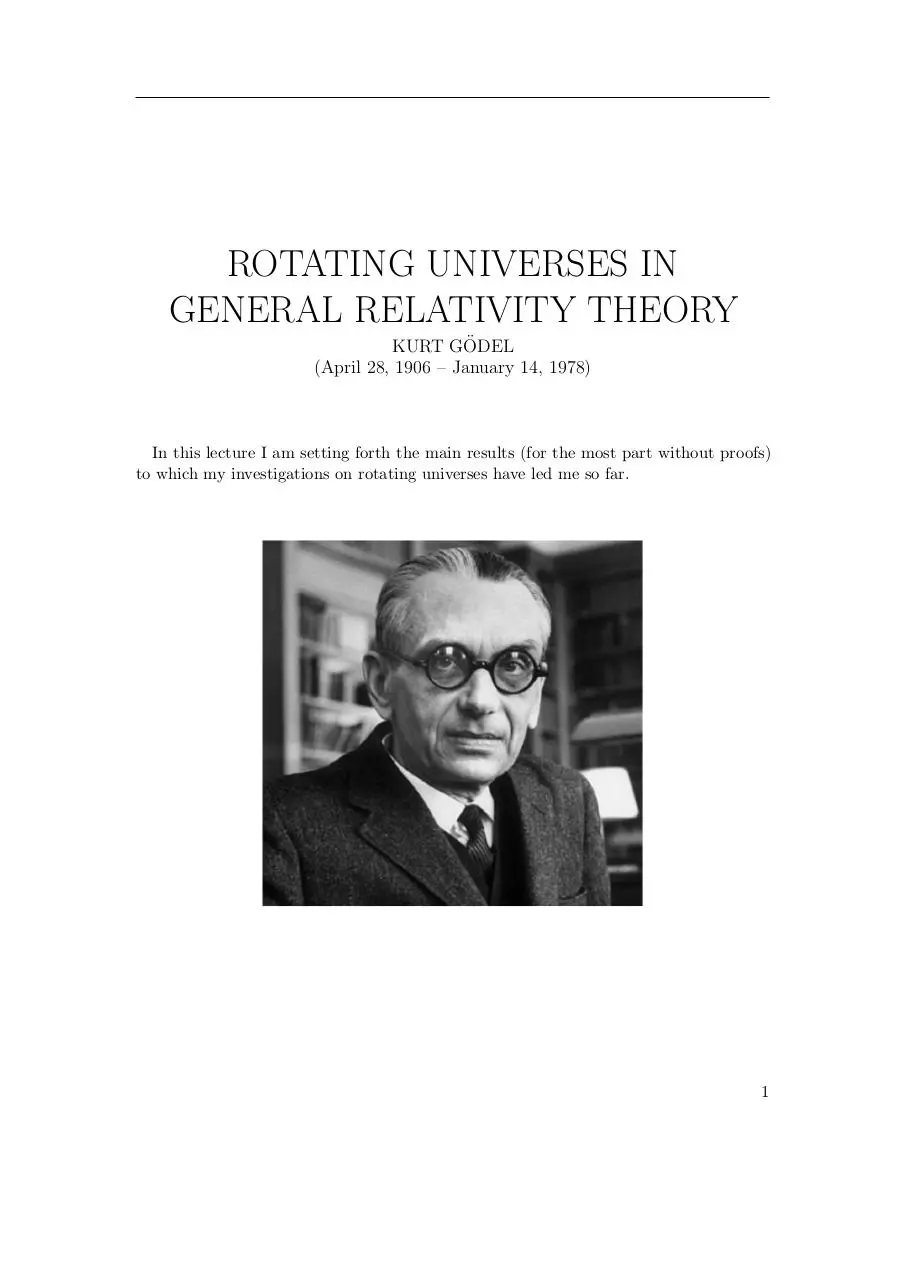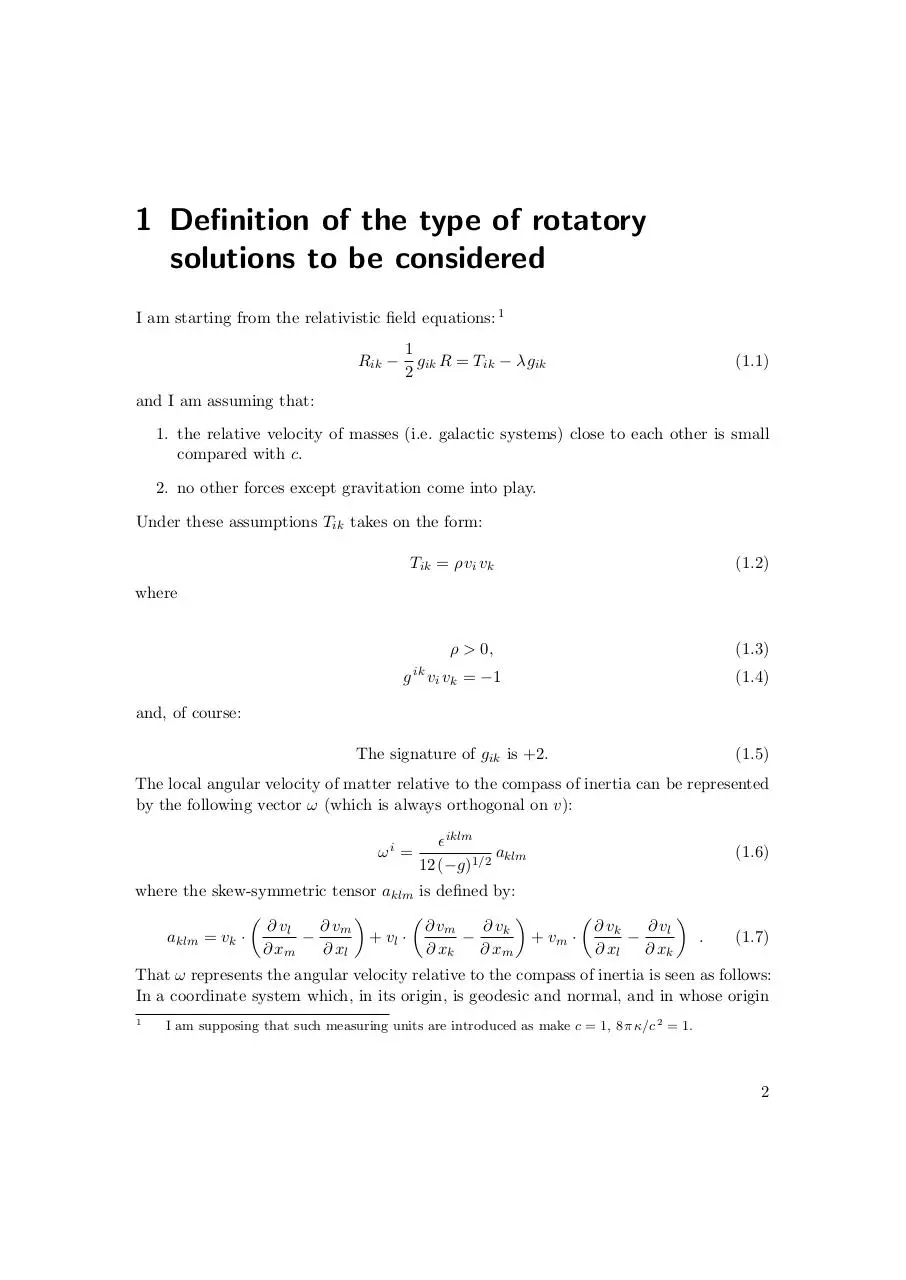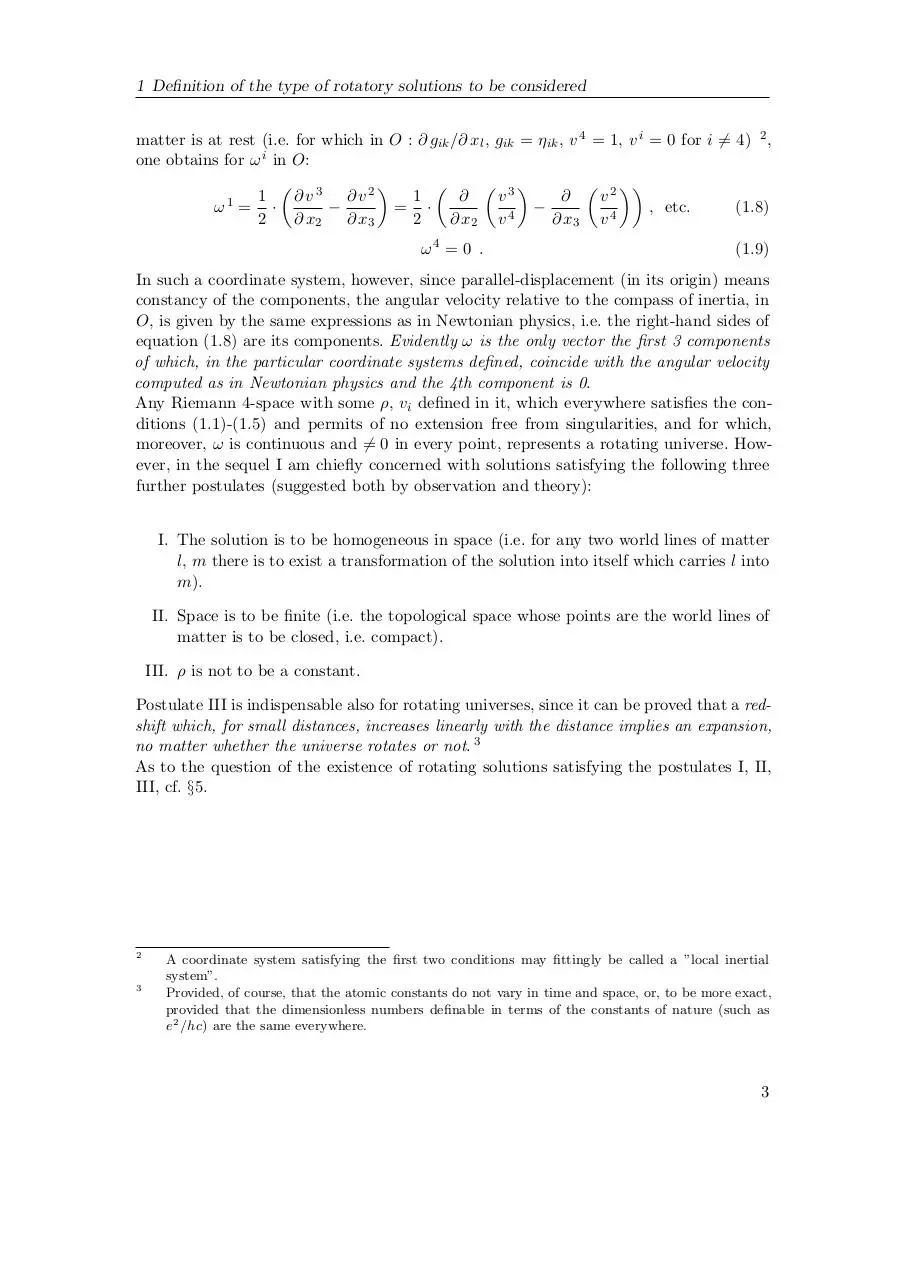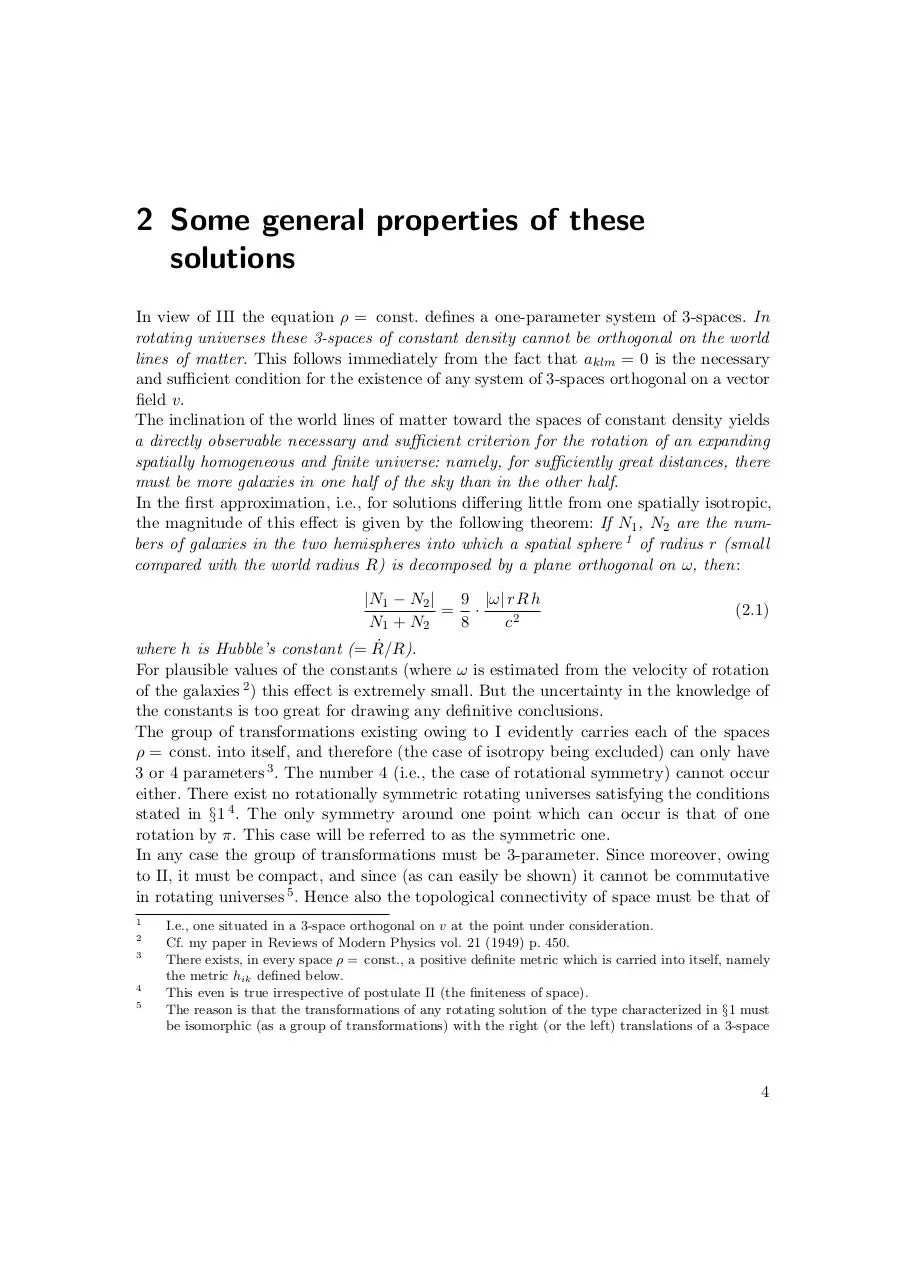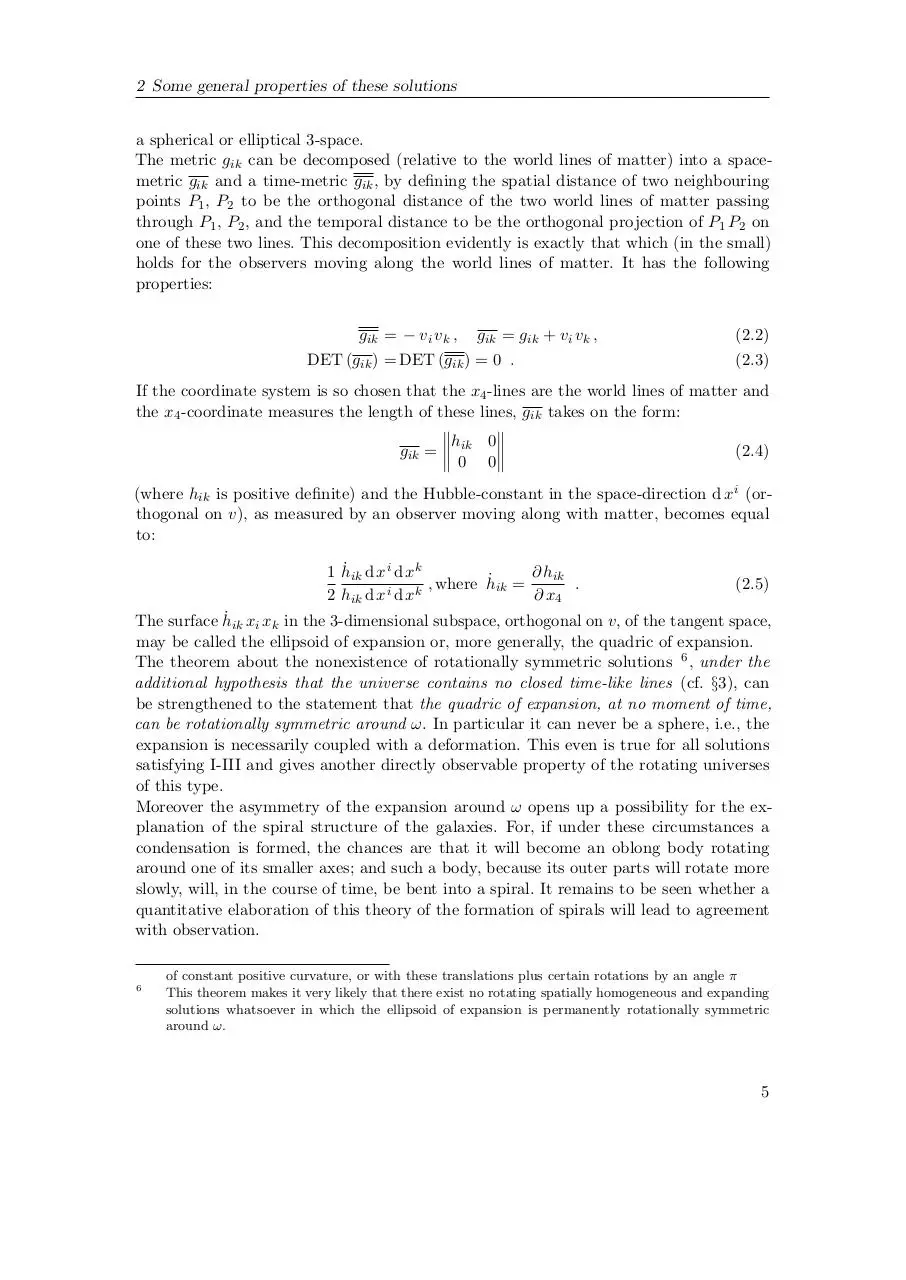Kurt GÃ¶del. Rotating Universes In General Relativity Theory.pdf (PDF, 283.92 KB)

### Share on social networks

#### HTML Code

Copy the following HTML code to share your document on a Website or Blog